/

### Archimedean copulas

A copula is a specialised form of multivariate probability distribution that has uniform marginals (technically the copula is the cumulative distribution function of such a distribution). In practice, researchers tend either to use:

(a)    Copulas implicitly derived from well-known multivariate distributions, e.g. the Gaussian copula (which is the copula applicable to multivariate normal, i.e. Gaussian, distributions) or the t copula (which is the copula applicable to multivariate t distributions); or

(b)    Simpler explicit copulas that depend on a handful of parameters, often just one.

Any multivariate distribution can in principle be the source of a copula; all we need to do is rescale the individual random variables so that they each have uniform marginal distributions. Copulas derived in this manner are called implicit copulas. The Gaussian copula is an example of such a copula.

Simpler copulas tend to be explicit, i.e. to be specifically defined by reference to the form of their copula. Examples include the following (whereis the dimension of the multivariate distribution):

 Copula Name DistributionName used to access this copula in the Nematrian function library Parameter Clayton Clayton Copula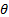Comonotonicity Comonotonicity Copula - Countermonotonicity Countermonotonicity Copula -* Frank Frank CopulaGeneralised Clayton Generalised Clayton Copula,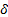Gumbel Gumbel CopulaIndependence Independence Copula -

* The countermonotonicity copula is only defined for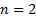.

Many of the copulas tabulated above are Archimedean copulas. These are copulas which take the form: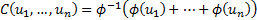Here,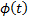is called the generator function of the Archimedean copula and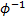is its inverse function. In some instances, (e.g. when we extend the range of the Clayton copula to include values forto include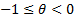) we need to replacein the above by the pseudo-inverse,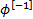. Suppose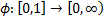is a continuous strictly decreasing function that maps the interval 0 to 1 onto the non-negative real line and has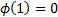and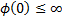. Then its pseudo-inverse is defined as: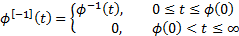A strict generator is one that has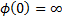, i.e. where the pseudo inverse function is the inverse function.

A bivariate copula (i.e. with) defined as above is a copula if and only if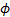is convex. A necessary condition for a multivariate Archimedean copula (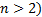defined as above to be a copula is that the generator is strict. It also needs to have its generator inverse being completely monotonic. A decreasing function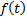is completely monotonic on the interval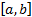if it satisfies: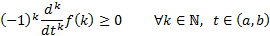A bivariate Archimdean copula has a Kendall’s tau,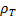, as follows: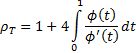Where the copula depends on just one parameter then this allows the parameter to be estimated from observed data.

The Archimedean copulas described above are exchangeable, by which is meant that they have the same structural form if we swap different dimensions around, since e.g.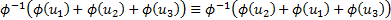etc.

Bivariate Archimedean copulas are often used in modelling applications. However, as the number of dimensions increases it becomes increasingly implausible that the ‘true’ dependency structure should be exchangeable across all possible variables simultaneously. A number of refinements have been proposed that include some but not complete exchangeability, see e.g. McNeil et al. (2005).